## January 23, 2007

### Scribe Post: Day 78

Hi everyone! This is Jann and I'll be your scribe for today.

Today, we practiced more antiderivative questions using "Integration by Parts".
Here we go...

1. Find the antiderivatives of the following:Q: How can we decide which function should be "u" (aka "f") and which one should be "dv" (aka "g' ")??

A: Good question! Today, we learned a new "rule". It's the...This rule is used to decide which of the two functions will be "f" and "g' ". This rule is followed from top to bottom (or left to right if you wrote it in that type of manner). For example, if an "algebraic" and a "trigonometric" is present in the problem, then "f"= "algebraic" and "g' "= "trigonometric". According to the rule, "A" comes before "T".

Moving on...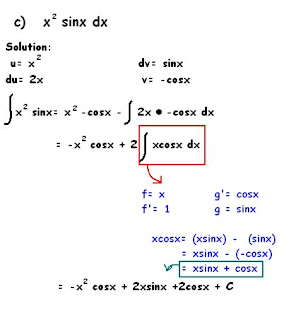Q: How come we did the "Integration by Parts" twice?

A: We did the technique twice because we dont know the antiderivative of "xcosx". We have to use the technique again to find the antiderivative of this expression.

Then, we answered some trig problems...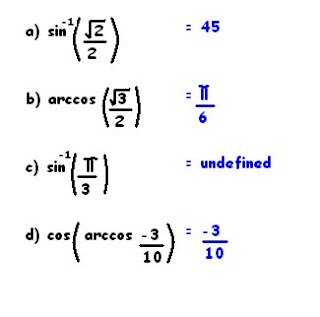Note: "Arc(cos or sin)" is the same as "sin or cos"^-1.

The answer to letter "c" is undefined because the value is greater than 1. The answer to letter "d" is -3/10 because cos and arccos cancel each other out.

Finally we looked at this graph...This is the graph of y= Sine. It is fixed in the domain, (-pi/2, pi/2). By doing this, the graph of sine is now a "One-to-one" function. It means that each input has 1 and only 1 output.

Refresher:

1. Vertical Line Test- this test is used to determine if the graph is a function or a relation. If the line test touches the graph more than twice (1 input has 2 or more outputs), then it is a relation.

2. Horizontal Line Test- this test is used to determine if the inverse of a function is a function or a relation. If the line touches the graph of the parent function more than twice, then the inverse of the graph is a relation.

I hope I covered most of the things we did for this class. XD

Next scribe will be Danny.

## January 21, 2007

Hi everyone! On friday's class we learned all about the method of substitution.

There are 3 main steps to this method:

----------------------------------------------

.

1. SUBSTITUTE:

-you let the inner function g(x) = u
-differentiate that inner function and let it = du/dx
-we solve for du by multiplying dx to the other side (cross multiply):
eg.
du = 3
dx
du = 3dx
-substitute u and du in original given integral.

2. ANTIDIFFERENTIATE:

-antidifferentiate the new integral that consist of u and du.

3.RESUBSTITUTE:

-substitute g(x) the inner function in for u to get your final answer, an antiderivative.

-------------------------------------------

-------------------------------------------

The following examples we did in class will show and explain this method:

-------------------------------------------
1) the integral of sin (3x) dxClick--> on pic so it's easier to read

*DON'T FORGET + C

.

--------------------------------------------

2) the integral of 5e^5x dxClick--> on pic so it's easier to read

*DON'T FORGET + C

--------------------------------------------

3) the integral of (x^3+2)^4 ( 3x^2) dxClick--> on pic so it's easier to read

Answer: (x^3+2)^5 / 5 + C

*DON'T FORGET + C.

.
----------------------------------------------

3) the integral of (x^3+2)^4 ( x^2) dx

- similar to question #3 but WITHOUT the 3 in front of x^

Click--> on pic so it's easier to read

Answer: (x^3+2)^5 / 15 + C

*DON'T FORGET + C.

-----------------------------------------------

.

4) the integral of (x / √x+7 ) dxClick--> on pic so it's easier to read

Answer: 2/3(x+7)^3/2 - 14(x+7)^1/2 + C

*DON'T FORGET + C.

----------------------------------------------
HOMEWORK: 7.3 Exercises --> odd #'s

-----------------------------------------------------------

The next scriber is MARK =]

## January 18, 2007

Well this chapter is about Finding Antiderivitives. By the end of this unit we'll have a bag filled with a bunch of tricks that will come in handy. :D

Remember in general,
If g is any particular antiderivitive of f, then we write
S f(x) dx = G(x) + C

Today we basically did seven questions on the board and discussed about how finding the antiderivitve of a function was not that easy than finding it's derivitive.

Sorry everybody but I'll finish this later tonight. My internet just connected. :(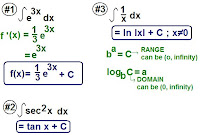Homework: EXERCISE 7.1 AND 7.2 ODD NUMBERS

The scribe for tomorrow is... Katrin

## January 17, 2007

### Scribe Post: Day 74

Hey guys!

The first thing we did in class was discuss our picture assignment. We were assigned to capture a scene that depicts "integrals", and were given since the beginning of the winter break to do it. We all found it tough, so much so that we asked Mr. K to change the theme. So he did. The new theme for our photo assignment is related rates.

We are to take a picture of something that shows related rates. The photo must be uploaded on Flickr, tagged "apcalc06", "related" and "rates". We are given over a week to do this!

After that, we continued writing our test. Mr. K originally gave us 10 minutes to do it, but we ended up taking all but 5 minutes of the class, so we didn't really start anything. We were just reminded that when antidifferentiating, we use the following formula for polynomials:We add "+ C" at the end of whatever terms we get, because we know that unless we're given specific points, we don't know which of a family of functions the antiderivatives (or derivatives) are talking about. Also, there are functions that just aren't antidifferentiable (I don't know if this word exists). Sometimes we just have to guess and check. An example is e2x. That's pretty much it.

Homework: 7.1 do odds from 1-17

Next Scribe: Crystal

## January 16, 2007

### bob

well all i can say is that this unit was very hard. i think it is the hardest so far. i find almost everything complicating lol. i know im not ready for the test but i can only blame myself for that. next time ill try my hardest not to procrastinate.

## January 15, 2007

### BOB

I forgot about BOB =O. Like everyone else said, this unit went in depth in integrals. The part where we had to teach a buddy in class about what we learned the day before was EXTREMELY helpful! It made me listen harder, and do you work. I was excited about it. I think I did a good job and teaching my buddy. At least, I think I did. When problems were given to us on the board, sometimes I had no idea what to do and freaked. But when Mr. K explained it to me it was like "OHHH." It all just made sense. I missed the calculator part because I wasn't in class, but I figured it out. On our pre-test today, the part that got me was the last bit. Where it had to do with the intervals and all. I keep forgetting how to follow that specific way we have to write... GAHH...

Some thoughts =D

### BOB

Hi! Once again, here we are, a unit's about to pass, and we're trying inch by inch trying to get to our prized goal, June.

What's different about this unit for me, I guess, is that I'm having a little bit more fun. I was on the bus to school one day, when I asked myself, "Why was calculus invented??!! WHYY?!!," when a thought came to mind. Maybe my attitude towards Calculus affects my learning, and therefore my marks in the class. If I don't treat Calculus as an enemy, maybe I'll be better at it. When I thought of this, I remembered my marks in Chemistry and Physics. They were a lot different ;). The difference between the two was that I thought of Chemistry as a fun subject while in Physics, even before I learned anything, I already thought it was going to be hard and boring. My mark in Chemistry was much higher, I think partly because I was motivated to do all the homework, not actually treating it like "work".

The day this happened, I told myself Calculus should be my friend, and from then on I've been having more fun in Calculus. That was an "ah hah moment" for me. Hopefully the mark I get on the test will reflect the fun I've been having =).

### BOB

Another BOB, yet another unit down. This one seemed to be the quickest of them all, mainly because the main thing, Integrals, we already learned from before. The only difference is we further expanded our knowledge of integrals by learning the Fundamental Theorem Of Calculus part 2, revisiting FTC part 1 and using it more, and our definition of an integral grew to a more distinct descriptive definition. Out of all the units so far I found this the most easiest to pick up and fastest to understand. I feel as if I was able to grasp the concept of using the different things we learned to solve Integrals, then again I can be wrong. I'll have to wait and see how the test turns out for me before I can be for sure. The pre-test was a bit challenging but only because I came not prepared and nervous. I must learn to calm myself down, think, and focus to the best of my ability. When I went into the group discussion, I noticed that the solutions to most of the questions I already knew, I just wasn't able to focus and think straight to get the answer. Hopefully things are different tomorrow for me as well as for everyone. Good luck to all on the test and to everyone with their exams. Study hard. Take it from me, I've been there before and I'm telling you no matter how smart you may be and no matter how easy school has been all your life, nothing is going to prepare you for what you're about to experience. Nothing but hard work and studying that is. =P

### Blogon the Blog #6

In today's class we got a pre-test for our current unit on Intergrals. I'm quite pleased at where I am at with this unit compared to the other ones where I much rather not talk about =). I am much more familiarized and understanding of these concepts. For the pre-test I got a majority of the questions right on my own, and the other questions I learned to do from the correction and learned that I made only few mechanical mistakes as an error. The group discussion certainly made the difference though! The biggest trouble for me in the units is having to visually picture (without having to really think about it)what's going on when I see the intergrated equations over an interval. That can be overcomed by doing more repitition of problems, and I will look over the review tonight and tomorrow morning to help me with it. Well goodluck on the test you guys! And goodluck on the upcoming exams later in a few weeks, too =|.

P.S. guys don't forget to label your posts =)

### BOB ---> integrals

WHOA is this BOB 6? This chapter was pretty easy to understand, which I'm really happy about because I understand it!! Well most of it, there's always something that I leave out but after a few hours of studying I'll feel confident enough to walk into that class tomorrow morning and write that integrals test! I just have to do the review problems and read my notes, and maybe make some more legible notes... The pre-test today was okay for me. Okay meaning I understand most of it but didn't get everything right. I did learn it though as we went through the answers so brownie points for me =). I hope the class does really well tomorrow! 100% to all! I'm listening to music right now. It puts me in a good mood, which makes studying muuuch easier. I wonder what you guys do to study. Well, whatever you do I hope it really helps. YAY INTEGRALS. XD

### integrals BOB

This unit faces a couple of problems, especially near the start. When we got to drawing the underlying graphs of an integral i was confused at first. This unit test is going to be really tough for me, i tried to do my homework and at least get a a couple problems down for practice. Hopefully tonight i will get the concepts we learned in this unit down. THeir short and sweet, but applying them is the hard part. Good luck everyone =\.

### BOB #6

This unit on Integrals was much more complex than the unit we did on Integrals before. We learned all about the first Fundamental Theorem of Calculus and the 2nd FTC. I thought that the first 2 excercise were pretty basic because I was able to answer the questions easily. Though for the last 2 excercises I had to really think hard and I had to even look at my old notes to get the question done. I needed to look at my old notes for especially questions about critical #'s, concavity, global mins and maxs, increasing/decreasing, etc. I forgot those concepts so then I needed to look at my old notes. After the pre-test I felt relieved that I knew how to do some of the questions. On the other hand, I still need to look at my notes and do more questions so that I am prepared for the test tomorrow... Good luck guys! =)

### BOB

I think I'm ready! Today's pretest went well for me, better than expected actually. And I'm doing well on the supplementary problems too. One thing I was confused about earlier was the calculator stuff, but we went over that in class and after that I found it more convenient to use the calculator than solve the problem by hand. But I think that was a good thing, because at first I had to do the questions by hand, instead of relying on technology to do the hard stuff. It prevented me from being lazy :)

I was thinking earlier about how this course is progressing, and I realized that we really learned a few main things- about derivatives and integrals. The whole course is based on these things, and each chapter we're just looking at them in a different light, and in more depth, and connecting them in a different way. It's unlike previous courses where we cover such a broad range of topics. So I guess that means we get a good overall understanding of these concepts. I think it's great to be able to look at these things in many different ways - I think we're learning to connect what we learn to other concepts better. Which is definitely a good skill to learn. Well, good luck on the test tomorrow everyone!

### BOB

In this unit, we talked about Integrals. We learned how to integrate a function and the different principles involved. We also talked about the 2 parts of the Fundamental Theorem of Calculus. Even though this unit has been really short, I learned a lot about integrals. The only part I had trouble with was calculating the area in a given region. I have to focus more on this idea before I go to bed. I hope everyone will study extremely hard for the test. XD

### ChallengeLinger mentioned:

Things are happening so fast.. new year, new unit. And, we're going on a field trip.. woohoo!!!!

Just how will you meet those challenges?!

## January 14, 2007

### BOB

So when I walked into Friday's class and heard that Mr. K was going to give us a lesson in five minutes, I felt relieved. Then, when he put the questions up on the board, I was stunned. I didn't know what to do, and it seemed that none of us did. But that was easily fixed ;) After going over the questions in depth, (and asking a lot of questions) I felt more at ease. The important thing to remember is the Fundamental Theorem of Calculus Part One and Two. And as long as we do the homework and get practise, we should be okay for the test =)

We're finally going to solve the amusement park problem... it makes me feel good because we can finally apply our learning to one huge question. It was only a couple of months ago, when Mr. K told us that we'd get here... and we're here now. Things are happening so fast.. new year, new unit. And, we're going on a field trip.. woohoo!!!! Math has been a great (although challenging) adventure so far, I can't wait for the rest of it..

Speaking of which, I shall go study. I'm still frustrated about my scribe post, because I have to make a lot of changes and I wasn't quite done explaining things. Nonetheless, I can't do anything about it for now, so I shall use my time to study before it's too late. GOOD LUCK TO ALL, and sorry again about the post.. I promise I'll fix it when I can.

### Scribe Post: Day 72

Hey guys!!! Even though it was super duper cold out, we still had school on Friday. Since Charlene didn't make it to class, I volunteered to take over. Sorry I put this up late. I tried making the post on Friday, and when I came back to edit, the window just kept closing. So I had to delete that one and start over. Anyway, here comes a whole lot of math!!!

First of all, Mr. K gave us a five minute lesson on what we were learning:We then had the following questions on the board:
The first one being; Find the area between f(x) and g(x).

And the third;SUMMARY:

• always figure out which function is on top
• find the roots by letting the functions equal each other
• define the integral that you are looking for
• solve the integral

We started the fourth question, which was about the amusement park. Its super long, and looks like its a lot of work, but we're finally going to solve it, woohoo =) Since we didn't finish, I will add it after tomorrow's class.

Speaking of which, TOMORROW is the PRE-TEST and TUESDAY is the TEST.

With that being said, HMWK for the weekend is to finish 6.4 odds and Supplementary Problems.

Tomorrow's scribe is CHARLENE =)

## January 11, 2007

### Scribe Post: 70

Hey everyone, sorry for the later post, but it's up like I said it'd be =]. Didn't know I was scribe for this class, but here's what best I could explain it from off the top of my head.

Note: for the following problems... [ ba ] should be read as "the intergral over the interval from a to b" or as pictured like this...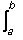1. Find g'(x):

a) g(x) = [x0] sin(t) dt
g'(x) = sinx
- We get this answer because we know and committed to memory as to what the antiderivative functions are for trig. functions which we learned are also 'transcendental' functions (don't mind the big word, isn't much useful) and we get -cosx. Then we use the Fundamental Theorum of Calculus Part 1... [ ba ] f'(t) = F(b) - F(a) because we're trying to find the definite intergral because when it is over an interval, we're looking for a number. And when there is no interval, then it turns out to be an indefinite integral.
- Using the FTC-part 1, we get g(x) =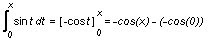.
- Simplified g(x) = -cosx + 1.
- Then we simply differentiate g(x) for g'(x)

From this problem, we find out that the derivative of the intergral function, is the same as the underlying function of the intergral. This is true for all functions and is interpreted through the FTC-part 2...The reason why the variable changes is because, we are only looking and changing x as the other variable t only changes as x does.

b) g(x) = [x0] √(1+t3) dt
g'(x) = √(1+x3)
- Using the FTC-part2. (See the pattern?)

c) g(x) = [3x²0] sin(t) dt
g'(x) = sin(3x2) · 6x
- We interpret this as a composite of functions, and then use the chain rule as we normally do to differentiate these functions...
g'(x) = f'(g(x)) · g'(x)

d) g(x) = [sinx0] √(1+t3) dt
g'(x) = √(1+sin3x) · -cosx
- Using FTC-part 2 and the chain rule law.

2. Graph of f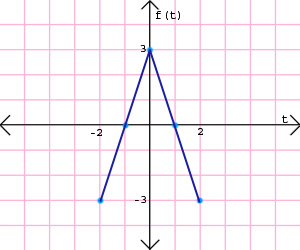g(x) = [x0] f(t) dt

a) Find g(1), g'(1), g''(1)
g(1) = 1.5
g'(1) = 0
g''(1) = -3

- g(1) is found by finding the sign area under the curve from 0 to 1, (f(1)·1)/2 'because it's a triangle.
- g'(1) is found by reading straight off from the graph, as the FTC-part 2 proves that a derivative of an intergrated function is just its underlying function.
- g''(1) is found by finding the slope of the tangent line at g'(1)

b) For what values of x on the interval (-2,2) is g increasing? Explain your reasoning.
(-1, 1)
Since g'(x) = f(t), we just look at where ever it is positively valued on the graph.

c) For what values of x, on the interval (-2,2) is g concave down?
(0, 2)
Since g'(x) = f(t), we just look at where ever its slope is negatively valued on the graph.

d) Sketch the graph of gThis is just one of the possible graphs for g as it is interpreted as a parent function derived from its derivative.
The graph of g'(x)=f(t), and the derivative only tells you the shape of the parent graph not where it is vertically. "If you were given a point on the graph, you would be able to position it, but if not, there would be infinitely many answers. (e's correction)" I got this answer by looking at the derivative and second derivative for increasing/decreasing and concavity characteristics. Aswell as integrating the function from -2 to 2, to find the total change for more accuracy. Using different intervals when intergrating will give you the same shape of the graph but it will be positioned different vertically.

Take care everyone!

Next scribe will be Charlene Linger, yay for the weekend.

## January 10, 2007

### scribe post

What did we do in class today?

For those of us who were writing the ELA provincial exam yesterday, today in class we buddied up with the students who were present in class and went over the questions of functions that required the use of integrals to solve. We pretty much did this for the entire class because some of us (me) didn't understand why the graph looked the way it did. Eventually I had one of those " lightbulb goes on moment " and figured it out.

Mr. K reviewed yesterdays class and explained everything and stressed the importance of understanding the domain of the functions. Which Suzanne has nicely explained in her scribe post (the one before this one).

Referring to the prior question in Suzanne's post, we learned about the domain of g(x):We also have this question qhich we did not complete but will probably go over tomorrow in class:A) What is the domain of g?
B) For what values of x does g'(x) = -1
C) Sketch the graph of g over it's entire domain

h m m . . . I sort of wished I had some math jokes but I don't =(

H o m e w o r k : Section 6.3 all the odd numbers

N e x t s c r i b e : manny

## January 09, 2007

### Scribe Post

Accumulation Functions Continued

For all of you guys who wrote the provincial English exams today, I hope you did well. We covered a lot in class today so I STRONGLY RECOMMEND YOU READ THIS.
As you probably remember, yesterday we looked at the graph of a function f(t). At the end of class we were given five integrals, and were asked to draw the corresponding graphs. We spent the class going over the answers to these.

First of all, here is the graph f(t) and the given information, which the whole class was based around.The graph of y=f(t): Is defined on the interval [0,4]
Has odd symmetry around the point (2,0)
On the interval [0,2], the graph is symmetric with respect to the line t=1

In yesterday’s class, we examined the graphs created by examining integrals on intervals, all using the function f(t). We discovered that f(t) was the derivative of all the different integrals we looked at. In other words, the graphs we created were all parent functions of f(t). In today’s class, we did the same thing, except that we had to do different transformations on the graph of f(t) and THEN find the graph of the parent function using the new graph.

I’m going to show the transformed graph for each question, followed by the graph of the parent function obtained from that graph.

1) Has somehow disappeared.. I'm very confused. Oh well. Anyway, the first question's basically what we did yesterday, no transformation involved. It just has the interval from x to zero, so in other words it the widths dx are negative, therefore the sign of the areas changes. The handy diagram below shows why. SEE YELLOW BOX. That's the thing to remember.OH I FOUND IT! So.. never mind, here's the first question.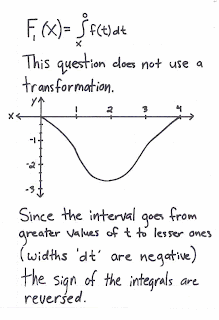2)3)If you were to look for the derivative of g(h(x)), you would use chain rule. So you would get
g'(h(x))*h'(x)
= f(2x)(2)

4)5)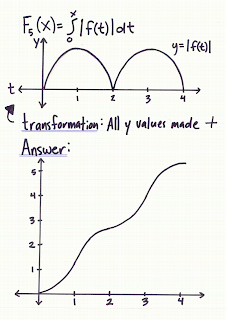And that's about all we did. Sorry this is up so late but the closer I get to a computer it seems the more technology-impaired I become. Oh right, and the next scribe is... Anh.

## January 08, 2007

### Peter Donnelly

Oxford statistician Peter Donnelly explores the common mistakes humans make in interpreting statistics, and the devastating impact these errors can have on the outcome of criminal trials.

Click on the picture. (22 min. 6 sec.)## January 07, 2007

### Scribe Post: Day 66

Well here I am almost the end of winter break and finally doing my scribe post. Reasons for me taking so long:

1. Basically the first week of the break I was sick and when I was getting better I'd get sick again =S
2. After that I was unable to find the sheet of notes I had jotted down during that class
3. Lastly, when I did find it I was already in that "winter break relaxed" type of state and became lazy to do it =S

If I hadn't got sick this post may have actually been up in the first few days of the break, now unfortunately I'm putting it up AFTER Christian's scribe post for....the day AFTER this class =S Well anyways I'm going to try and put together what I can remember from my notes and from the class.

We started a new unit, Chapter 6: Integrals. However before that we were debating over a question from the test on the past unit. After several minutes of debating we talked about integrals. Discussing on what the definition of an integral was we came up with these things:

- sign area under a curve
- antiderivative
- there are two types of integrals, indefinite and definite integrals
- indefinite integrals represents a family of functions with the same derivative and a vertical shift
- definite integrals represents different numbers.
- to find an integral you can use Rieman's Sum (left hand sum, right hand sum) although it won't give you an exact value, divide to sub intervals, find area under curve using rectangles.

Mr. K mentioned how we learned the first part of the Fundamental Theorem of Calculus and how we were going to learn the second part of it in this chapter, at least I think so from my notes? =S

The rest of my notes are extremely hard to read (I got to learn how to write faster and more clear when im writing fast =S) but there's one integral I wrote down because he had it on the board that he said he'll show us how get in a later unit, unfortunately it's too messy for me to put it down onto this post so if anyone has it, please comment so I can add it.

We learned the Constant Multiple Rule:Basically to make the first integral easier to do, you bring out the 2 and work with the basic integral, this is the Constant Multiple Rule I think? =S

To top off this horrible post, at the corner of my sheet of notes in capital letters it says" NEED TO KNOW ANTIDERIVATIVE TO FIND INTEGRAL" that has to be important cause it was in capitals. Well as you all know Christian was the next scribe and yes I hope you all enjoyed your winter break see you all tomorrow!

## January 06, 2007

### Scribe Post: Day 67

Hello everyone! It's Christian, and I was the scribe on the last school day of '06 =(. As a summary, we didn't really start anything new. We just reviewed some things that we learned in previous classes.

Integrals As Signed Areas Under a CurveGraphically speaking, an integral is the signed area under a curve. So, from the interval A to B on the graph above, the highlighted region is the integral.This graph is the same as the one up there, except that the axes are now labeled.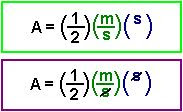To integrate on the interval A to B, we must find the area of the red triangle. If we do that, we'll end up with an equation similar to the one in the green box. Don't mind the "1/2". What's important to note is that if we solve this equation, the s's will cancel, and we'll be left with the unit m, as seen in the purple box. As we know, "metres" is a unit that indicates "position", d. The parent graph of a velocity-time graph is a position-time graph. YAY! This shows what we learned about integrals: that they are signed areas under a curve, and that they are the total change on a parent function.I'll explain the last concept we learned as quickly as possible. The graph above is, again, the same as the ones before, except that the integral from A to B is now divided into a blue region and green region. We learned that if we find the integral from A to Z and add that to the integral from Z to B, we'll end up with the same number as if we just took the integral from A to B.

That was the class!

Hey guys, hope you had a great winter break. Back to school now! No one skip the first day of classes! Can't wait to see all of you =)

I guess we're starting the new cycle, so the next scribe is... Crystal!